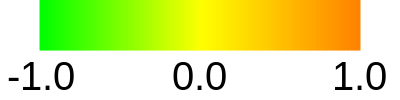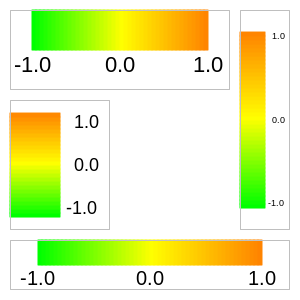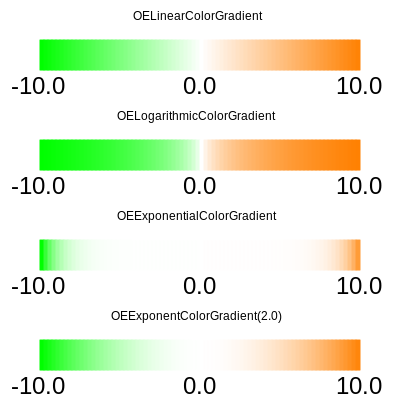void OEDrawColorGradient(OEDepict::OEImageBase &image,



image

The image on which the color gradient is drawn.

colorg

opts

The OEColorGradientDisplayOptions object that stores the parameters that determine how the color gradient is drawn.

The following code snippet shows how to use the OEDrawColorGradient function. The image created is shown in Figure: Example of using the OEDrawColorGradient function.

OELinearColorGradient colorg = new OELinearColorGradient();

OEImage image = new OEImage(400, 100);Example of using the OEDrawColorGradient function

The orientation (i.e., whether the color gradient is rendered vertically or horizontally) depends on the width and height of the OEImageBase object. If the width is greater than the height, then the color gradient is drawn horizontally; otherwise it is rendered vertically . See examples in Figure: Example of rendering the same color gradient into different dimensions.Example of rendering the same color gradient into different dimensions

The OEDrawColorGradient function can depict any color gradient derived from the OEColorGradientBase base class. See examples in Figure: Example of rendering various color gradients.Example of rendering various color gradients